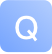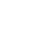/**

• Compute the probabilities of each value on the given [[DataSet]]
*
• @param x single colum [[DataSet]]
• @return Sequence of probabilites for each value
*/

private[this] def probs(x: DataSet[Double]): Seq[Double] = {

``````    val counts = x.groupBy(_.doubleValue)
.reduceGroup(_.size.toDouble)
.name("X Probs")
.collect

val total = counts.sum

counts.map(_ / total)``````

}

/**

• Computes 'symmetrical uncertainty' (SU) - a symmetric mutual information measure.
*
• It is defined as SU(X, y) = 2 * (IG(X|Y) / (H(X) + H(Y)))
*
• @param xy [[DataSet]] with two features
• @return SU value
*/

def symmetricalUncertainty(xy: DataSet[(Double, Double)]): Double = {

``````val su = xy.mapPartitionWith {
case in ⇒
val x = in map (_._2)
val y = in map (_._1)

val mu = mutualInformation(x, y)
val Hx = entropy(x)
val Hy = entropy(y)

Some(2 * mu / (Hx + Hy))
}

}

1 条回答写回答取消 提交回答

它使用n级并行：

def symmetricalUncertainty(xy: DataSet[(Double, Double)]): Double = {

``````val su = xy.reduceGroup { in ⇒
val invec = in.toVector
val x = invec map (_._2)
val y = invec map (_._1)

val mu = mutualInformation(x, y)
val Hx = entropy(x)
val Hy = entropy(y)

2 * mu / (Hx + Hy)
}

}
您可以在InformationTheory.scala及其测试InformationTheorySpec.scala中查看整个代码

2019-07-17 23:19:15
赞同 展开评论 打赏

23
0
0
12
0
0
11
0
0
12
0
0
10
0
0
113
0
0
74
1
0
65
1
0
74
0
0
52
1
0146214311311293810562295103